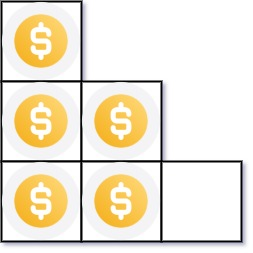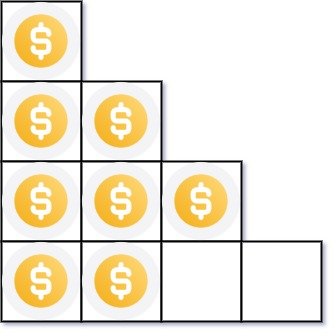# 441. Arranging Coins

Easy
You have `n` coins and you want to build a staircase with these coins. The staircase consists of `k` rows where the `ith` row has exactly `i` coins. The last row of the staircase may be incomplete.
Given the integer `n`, return the number of complete rows of the staircase you will build.
Example 1:Input: n = 5
Output:
2
Explanation:
Because the 3rd row is incomplete, we return 2.
Example 2:Input: n = 8
Output:
3
Explanation:
Because the 4th row is incomplete, we return 3.
Constraints:
• `1 <= n <= 231 - 1`

### 解題

Binary search + 總和公式 ： 1~k的和等於 k(1+k)/2
func arrangeCoins(n int) int {
if n == 1 { return 1 }
if n == 2 { return 1 }
if n == 3 { return 2 }
left := 0
right := n/2
res := 0
for left <= right {
mid := left + (right - left)/2
sum := mid*(mid+1)/2
if sum == n {
return mid
} else if sum > n {
right = mid - 1
} else {
res = mid
left = mid + 1
}
}
return res
}